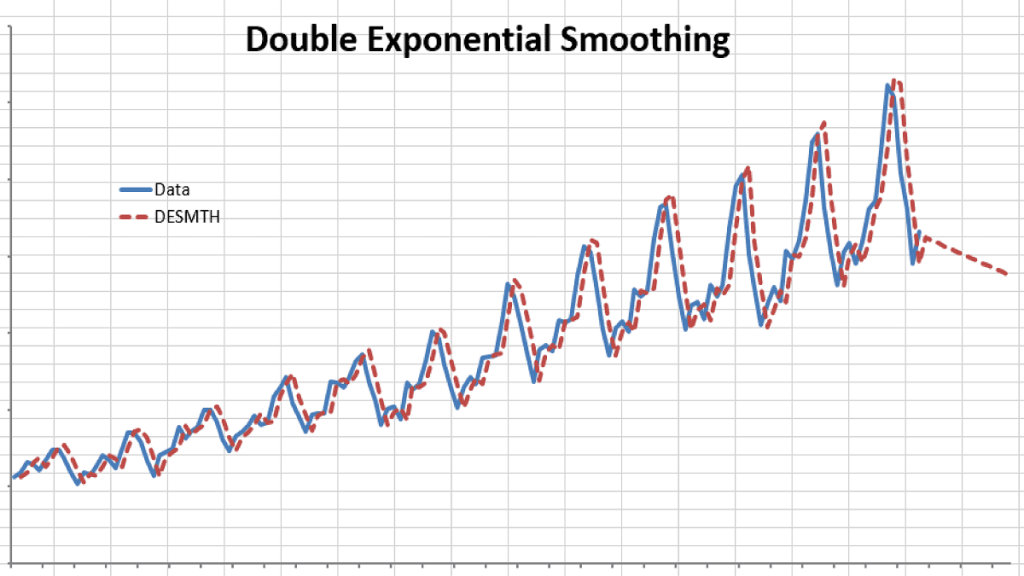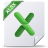# Holt’s Double Exponential Smoothing

Simple exponential smoothing does not do well in the presence of a trend, so several methods devised under the “Double Exponential” umbrella are proposed to handle this type of data.

NumXL supports Holt’s double exponential smoothing, which take the following formulation:

$$S_1=X_1$$ $$B_1=\frac{X_T-X_1}{T-1}$$ $$S_{t>1}=\alpha X_t + (1-\alpha)(S_{t-1}+B_{t-1})$$ $$B_{t>1}=\beta (S_t – S_{t-1})+(1-\beta)B_{t-1}$$ $$Y_t=\left\{\begin{matrix} S_t+B_t & t<T\\ S_T+m\times B_T & t=T+m \end{matrix}\right.$$

Where:

• $\alpha$ is the smoothing factor ($0 \prec \alpha \prec 1$)
• $\beta$ is the trend smoothing factor ($0 \prec \beta \prec 1$)

Example 1:

Let’s examine the international passengers’ airline data.

We chose an Alpha value of 0.9 and a Beta of 0.1. Please note that although double smoothing traces the original data well, the out-of-sample forecast is inferior to the simple moving average.

How do we find the best smoothing factors?

We take a similar approach to our simple exponential smoothing example but modified for two variables. We compute the sum of the squared errors; construct a two-variable data table, and pick the alpha and beta values that minimize the overall SSE.

## Files Examples